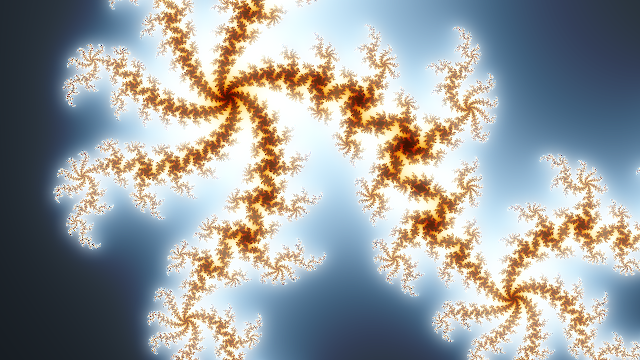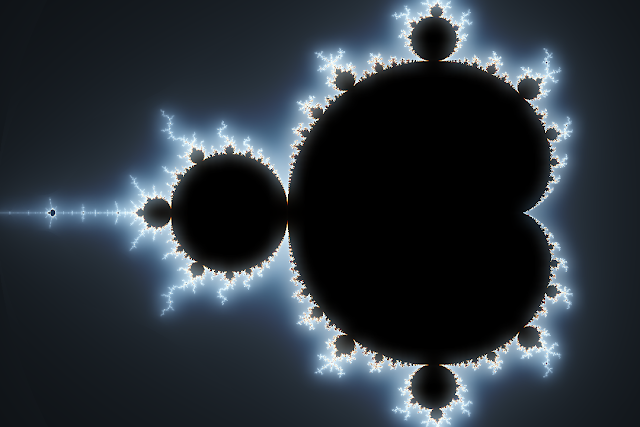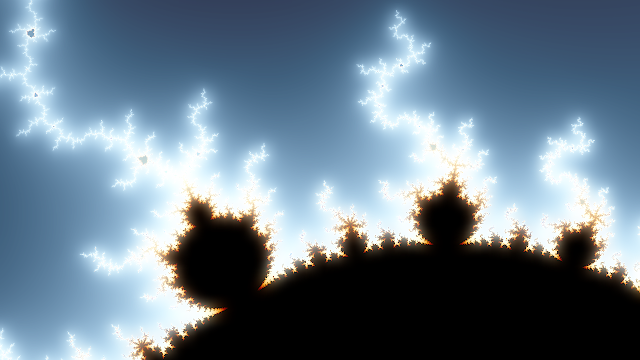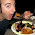## Tuesday, September 29, 2009

### Fractal Rendering on the GPU - Mandelbrot and Julia Sets in an HLSL ShaderI've been playing with HLSL shaders recently, and it occurred to me that the horsepower of the GPU could probably be harnessed to render fractals like the Mandelbrot Set. It turns out to be a perfect task for a shader, since it involves lots of completely localized calculations - exactly what graphics cards are good at.

A bit of web searching turned up the not surprising fact that I'm not the first person to have this realization. I found some nice code examples here and here. My version takes inspiration from both of these approaches, and adds a few tweaks of my own.

The image above is of the Julia set, rendered completely by the GPU. Below is, of course, the Mandelbrot Set.And another view of the Mandelbrot set zoomed in a bit:On my GeForce 8800GT, I can zoom and pan around at a rock-solid 60 frames per second. Here is a video exploring the Julia set. The warping you see is me transforming the seed used to render the set.

Here is the shader code I ended up with. It is pretty simple. I'm using the Normalized Iteration Count Algorithm to get nicely smoothed coloring. The parameters are set to sensible default values, but you will want to pass them in from your application and map them to some sort of input device. For rendering, simply draw a full screen quad using the shader. In my application I also gild the lily a bit and apply a bloom effect.

```int Iterations = 128;
float2 Pan = float2(0.5, 0);
float Zoom = 3;
float Aspect = 1;
float2 JuliaSeed = float2(0.39, -0.2);
float3 ColorScale = float3(4, 5, 6);

float ComputeValue(float2 v, float2 offset)
{
float vxsquare = 0;
float vysquare = 0;

int iteration = 0;
int lastIteration = Iterations;

do
{
vxsquare = v.x * v.x;
vysquare = v.y * v.y;

v = float2(vxsquare - vysquare, v.x * v.y * 2) + offset;

iteration++;

if ((lastIteration == Iterations) && (vxsquare + vysquare) > 4.0)
{
lastIteration = iteration + 1;
}
}
while (iteration < lastIteration);

return (float(iteration) - (log(log(sqrt(vxsquare + vysquare))) / log(2.0))) / float(Iterations);
}

float4 Mandelbrot_PixelShader(float2 texCoord : TEXCOORD0) : COLOR0
{
float2 v = (texCoord - 0.5) * Zoom * float2(1, Aspect) - Pan;

float val = ComputeValue(v, v);

return float4(sin(val * ColorScale.x), sin(val * ColorScale.y), sin(val * ColorScale.z), 1);
}

float4 Julia_PixelShader(float2 texCoord : TEXCOORD0) : COLOR0
{
float2 v = (texCoord - 0.5) * Zoom * float2(1, Aspect) - Pan;

float val = ComputeValue(v, JuliaSeed);

return float4(sin(val * ColorScale.x), sin(val * ColorScale.y), sin(val * ColorScale.z), 1);
}

technique Mandelbrot
{
pass
{
}
}

technique Julia
{
pass
{
}
}
```

1.amazing, beautiful.

2.Neat. On a somewhat related though all together different note I was looking into this the other day and thought you might be interested:
http://brahma.ananthonline.net/

Basically it is LinqToGPU. Seems pretty neat.

3.Thanks, pauliep!

Brad - Brahma looks interesting and strange. It was hard to find any concrete details on the website, though - short of actually downloading the code.

4.The images you posted look outstanding. Take a look at this for a web fractal generator:

5.Great job! I have problem when I try to compile my code. Is it possible to get the code sample?

6.Sorry, I don't have a clean code example.

Have a look at this example. It is one of the implementations I based mine on, and you should be able to easily modify it to work with my shader.

7.That’s the example I have implemented, the problem is that the Effect won’t compile. I get the following error.
"Cannot mix shader model 3.0 with earlier shader models. If either the vertex shader or pixel shader is compiled as 3.0, they must both be."
I must have a vertex shader vs_3_0. I know that this is issue for some graphic card. I got an ATI.

8.I implemented this colour smoothing technique in C#. I made an application simply it renders valuable constant Julia and Mandelbrot fractal. It is very beatiful, thank you...